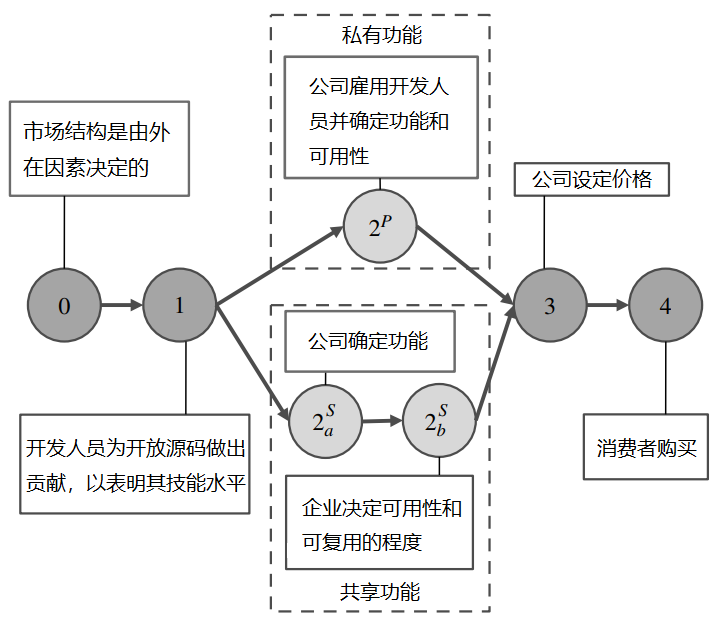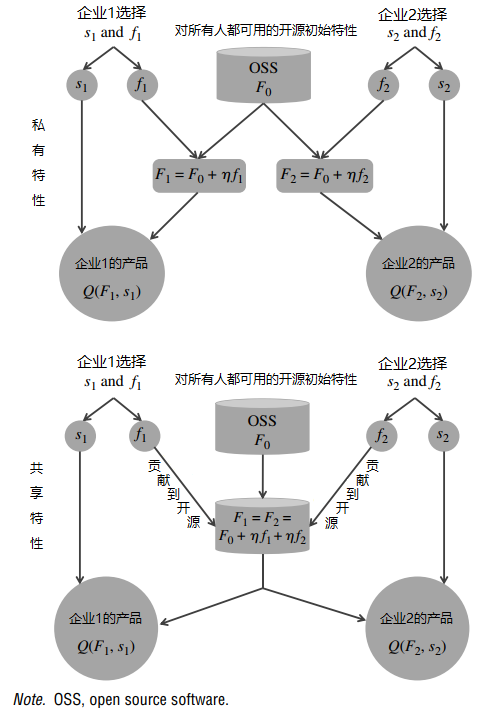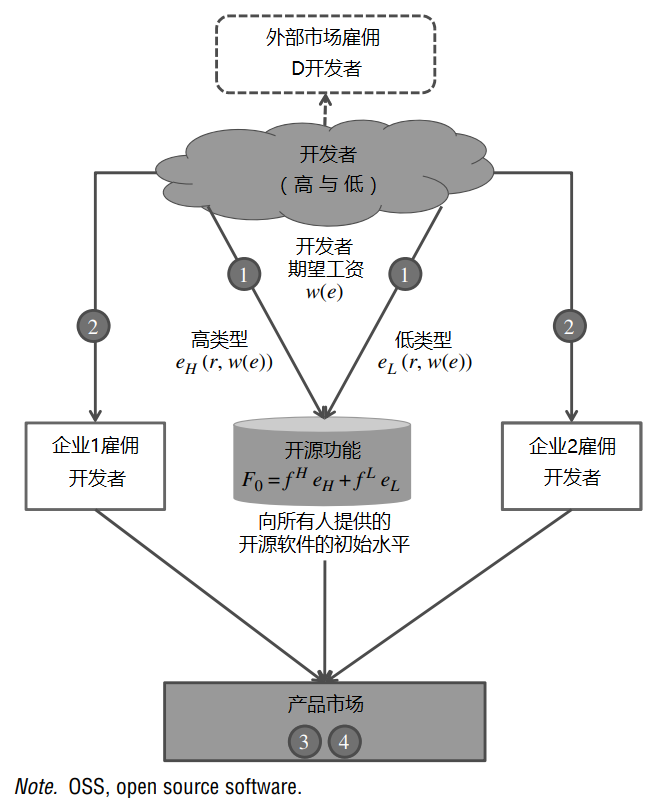# 【翻译】开源软件的竞争战略

• Vineet Kumar ：哈佛大学哈佛商学院，波士顿，马萨诸塞州，02163，vkumar@hbs.edu
• Brett R. Gordon ：哥伦比亚大学商学院，纽约，纽约，10027，brgordon@columbia.edu
• Kannan Srinivasan ：卡内基梅隆大学泰珀商学院，宾夕法尼亚州匹兹堡，15213，kannans@andrew.cmu.edu。

## 2. 开放源码产业

1. 功能：提供新的或增强的功能，扩展软件的基本操作。例如，与开源的 OpenOffice 相比，Sun 公司的 StarOffice 套件具有额外的功能，提供对不同文档格式的兼容性和支持。

2. 可用性和支持服务：提高用户有效使用产品现有功能的能力。可用性的增强通常采取非软件服务的形式，如在线帮助、技术援助、文档、包装和其他支持服务。6

## 3. 模式——私人和共享特征市场M 市场规模、异质性
$$C_L,C_H$$ 每种类型的开发者向开源贡献一个功能的成本
$$C_s$$ 开发一个单位的可用性的成本
$$\eta_H (=\eta),\eta_L$$ 高类型和低类型开发者的生产率分别为

$$p_j, q_j$$ j 公司产品的价格和质量，分别为
$$f_j, s_j$$ j 公司雇用的开发人员数量和可用性投资，分别为
$$F_j$$ 公司 j 的产品的特征数量
$$F_0$$ 由于开发者的贡献而产生的开放源码功能的数量
$$e_L, e_H$$ 低类型和高类型开发者对开放源码的贡献分别为
W(e) 开发者市场的工资，与 $$e \in \{ e_L, e_H \}$$

### 3.1. 产品市场

$u(q;\theta) = \theta q - p.$

$q = Q(F, s).$

${\partial^2Q \over \partial s \partial F} > 0.$$$q^P_j = Q(F_j, s_j) = [(F_0 + \eta f_j)s_j]^{1/4},$$ and $$q^S_j = Q(F_j, s_j) = [(F_0 + \eta f_j + \eta \delta_j f_{-j})s_j]^{1/4}$$.

### 3.2. 开发者市场\begin{aligned} &{e_t = \underset{e}{\arg \ max} \ w(e) - c_t e,} & & (1) \end{aligned}

\begin{aligned} {\begin{aligned} &(IC_H): w(e_H) - c_H ≥ w(e_L) - C_H e_L, \\\\ &(IR_H): w(e_H) - c_H e_H ≥ r, \\\\ &(IC_L): w(e_L) - c_L e_L ≥ w(e_H) - c_L e_H, \\\\ &(IR_L): w(e_L) - c_L e_L ≥ 0. \end{aligned}} & & (2) \end{aligned}

\begin{aligned} &{F_0(w) = f_0 e_H = \Psi(w(e_H) - c_H e_H ) eH.} & & (3) \end{aligned}

## 4. 平衡分析

### 4.1. 定价均衡

$m_1 = {1 \over M} (M - \theta_{12}) = {1 \over M} (M - { {p_1 - p_2} \over {q_1 - q_2} }), and$ $m_2 = {1 \over M} (\theta_{12} - \theta_{20}) = {1 \over M} ({ {p_1 - p_2} \over {q_1 - q_2} } - {p_2 \over q_2}).$

$p^*_1 = \underset{p1}{\arg max} (M -{ {p_1-p_2} \over {q_1 - q_2} }) p_1, and$ $p^*_2 = \underset{p2}{\arg max} ({ {p_1-p_2} \over {q_1 - q_2} } - {p_2 \over q_2}) p_2.$

### 4.2. 产品质量均衡

$$s^P_j = \pi^S_j M^2 \sqrt{\eta \over {c_s}^3 w},$$ $$s^P_j = \psi^Q_j M^2 \sqrt{\eta \over {c_s}^3 w},$$
$$f^P_j = \pi^F_j M^2 \sqrt{\eta \over c_s w^3} - {F_0 \over \eta},$$ {\begin{aligned} &f^S_1 = \psi^F_1 M^2 \sqrt{\eta \over c_s w^3} - {F_0 \over \eta}, \\ &f^S_2 = 0, \end{aligned}}
$$q^P_j = \pi^Q_j M \sqrt{\eta \over c_s w},$$ $$q^S_j = \psi^Q_j M \sqrt{\eta \over c_s w}.$$

## 5. 市场互动和比较

\begin{aligned} & &\xi^P(w) = &D(w) + M^2 \sqrt{\eta \over {c_s w^3} } D(\pi^F_1 + \pi^F_2) & & &\\\\ & & &-2 {w \over c_L} \Psi(w [1 - {c_H \over c_L} ]) - \Psi (w [1 - {c_H \over c_L} ]),\\\\ & &\xi^S(w) = & \underbrace {D(w)}_{External \ demand} \\\\ & & & + \underbrace {M^2 \sqrt{\eta \over c_s w^3} \psi^F_1 - {w \over c_L} \Psi (w [1 - {c_H \over c_L}])}_{COSS \ demand D = f_1 + f_2} \\\\ & & & - \underbrace{\Psi (w - [1 - {c_H \over c_L}])}_{Developers' signaling}. & & (4) \end{aligned}

(i) 共享特征市场上的工资低于 在私人特征市场上，$$w^S < w^P$$。

(ii) 在两个市场中，w 都满足以下属性：w 在市场规模 $$(\delta w / \delta M > 0)$$、信号成本 $$(\delta w / \delta c_H > 0)$$ 和技能水平 $$(\delta w / \delta \eta > 0)$$ 中是增加的；在可用性成本 $$(\delta w / \delta c_s < 0)$$ 中是减少的。

(i) 私人特征市场提供最低的质量水平。

(ii) 对于小的市场规模和低的信号成本，私有功能市场提供了最高质量的产品。

(iii) 共享功能市场的可用性比率 s1/s2 总是比私人功能市场的大，而私人功能市场下的功能比率 f1/f2 更大。私人功能市场的质量差异化比率 q1/q2 的捕获量比共享功能市场的要高。

(i) 在共享特征市场下，高质量的公司可以获得更高的利润。

(ii) 在所有市场条件下，共享特征市场的消费者剩余都高于私人特征市场。

(ii) 中的消费者剩余结果令人惊讶地具有普遍性和反直觉性：企业对开发者竞争的减少增加了对消费者的剩余。其理由是，企业开发的产品中使用的信号（开源功能）的效用，以及由于低质量企业对功能的搭便车而导致的开发者市场竞争的减少，都有利于消费者。因此，与鲍尔默的观点相反，即强制性共享许可是一个“附着在自己身上的癌症”，我们发现这样的要求可能对消费者和公司都有利。

## 鸣谢

• Abran, A., A. Khelifi, W. Suryn, A. Seffah. 2003. Usability meanings and interpretations in ISO standards. Software Quality J. 11(4) 325–338.
• Bagozzi, R. P., U. M. Dholakia. 2006. Open source software user communities: A study of participation in Linux user groups. Management Sci. 52(7) 1099–1115.
• Boehm, B. W. 1981. Software Engineering Economics. Prentice-Hall, Englewood Cliffs, NJ. Casadesus-Masanell, R., P. Ghemawat. 2006. Dynamic mixed duopoly: A model motivated by * Linux vs. Windows. Management Sci. 52(7) 1072–1084.
• Casadesus-Masanell, R., G. Llanes. 2011. Mixed source. Management Sci. 57(7) 1212–1230.
• Cho, I. K., D. M. Kreps. 1987. Signaling games and stable equilibria. Quart. J. Econom. 102(2) 179–221.
• Dedeke, A. 2009. Is Linux better than Windows software? IEEE Software 26(3) 103–104.
• Economides, N., E. Katsamakas. 2006. Two-sided competition of proprietary vs. open source technology platforms and the implications for the software industry. Management Sci. 52(7) 1057–1071.
• Economist, The. 2009. Open-source software in the recession: Born free. (May 28) http://www.economist.com/node/13743278.
• Fershtman, C., N. Gandal. 2007. Open source software: Motivation and restrictive licensing. Internat. Econom. Econom. Policy 4(2) 209–225.
• Fuller, T. 2003. How Microsoft warded off rival. New York Times (May 15) http://www.nytimes.com/2003/05/15/technology/15SOFT.html.
• Gartner Group. 2008. Gartner highlights key predictions for It organisations and users in 2008 and beyond. Press release (January 31), Egham, UK. http://www.gartner.com/it/page.jsp?id=593207.
• Goldman, R., R. P. Gabriel. 2005. Innovation Happens Elsewhere: Open Source as Business Strategy, 1st ed. Morgan Kaufmann, San Francisco.
• Handy, A. 2008. Red Hat tops list of corporate Linux code contributors. Software Development Times (September 18) http://www.sdtimes.com/link/32870.
• Hars, A., S. Ou. 2002. Working for free? Motivations for participating in open-source projects. Internat. J. Electronic Commerce 6(3) 25–39.
• IDC. 2009. Worldwide open source services 2009–2013 forecast. Report, IDC, Framingham, MA.
• InfoWorld. 2000. Open-source platforms: IBM invests almost \$1 billion in Linux. (December 18) 16.
• Jung, H. W., S. G. Kim, C. S. Chung. 2004. Measuring software product quality: A survey of ISO/IEC 9126. IEEE Software 21(5) 88–92.
• Lakhani, K. R., E. von Hippel. 2003. How open source software works: “Free” user-to-user assistance. Res. Policy 32(6) 923–943.
• Laurent, L. S. 2004. Understand Open Source and Free Software Licencing. O’Reilly, Cambridge, MA.
• Leppamaki, M., M. Mustonen. 2009. Skill signalling with product market externality. Econom. J. 119(539) 1130–1142.
• Lerner, J., J. Tirole. 2002. Some simple economics of open source. J. Indust. Econom. 50(2) 197–234.
• Moorthy, K. S. 1988. Product and price competition in a duopoly. Marketing Sci. 7(2) 141–168.
• Pal, N., T. R. Madanmohan. 2002. Competing on open source: Strategies and practise. Working paper, Massachusetts Institute of Technology, Cambridge. http://opensource.mit.edu/online_papers.php.
• Pressman, R. B. 2004. Software Engineering: A Practitioner’s Approach. McGraw-Hill, New York.
• Ramaswamy, V., F. Gouillart. 2010. The Power of Co-Creation: Build It with Them to Boost Growth, Productivity, and Profits. Free Press, New York.
• Riehle, D. 2007. The economic motivation of open source software: Stakeholder perspectives. Computer 40(4) 25–32.
• Roberts, J. A., I. Hann, S. A. Slaughter. 2006. Understanding the motivations, participation, and performance of open source software developers: A longitudinal study of the Apache projects. Management Sci. 52(7) 984–999.
• Sawhney, M., G. Verona, E. Prandelli. 2005. Collaborating to create: The Internet as a platform for customer engagement in product innovation. J. Interactive Marketing 19(4) 1–15.
• Shaked, A., J. Sutton. 1982. Relaxing price competition through product differentiation. Rev. Econom. Stud. 49(1) 3–13.
• Spence, M. 1973. Job market signaling. Quart. J. Econom. 87(3) 355–374.
• Thompson, D. V., R. W. Hamilton, R. T. Rust. 2005. Feature fatigue: When product capabilities become too much of a good thing. J. Marketing Res. 42(4) 431–442.

2. 开源软件的流行例子包括 Linux 操作系统、Firefox 浏览器、OpenOffice、Apache 网络服务器、SugarCRM 和 MySQL 等等。参见 http://www.sourceforge.net，了解基于网络的开放源码应用程序库。

4. 关于轶事，可以在 http://www.odesk.com/blog/2008/02/open-source-work-as-a-portfolio/ 和 http://blogs.techrepublic.com.com/opensource/?p=821 （2011 年 3 月 11 日访问）找到两个恰当的例子。

5. 参见 InfoWorld (2000).

6. 可用性也可以包括软件代码或附加程序，使界面更容易使用。

7. 基于开源的商业应用的详尽清单可以在维基百科上找到；http://en.wikipedia.org/wiki/Commercial_open_source_applications（2011 年 3 月 11 日访问）。

8. 一般来说，消费者不会从功能水平和可用性之间明显不平衡的产品中获益。Thompson 等人（2005）表明，购买过于复杂的产品的消费者会面临 “功能疲劳”，提高可用性可以帮助消费者有效地利用这些功能。

9. $$F_0$$ 在平衡状态下通过开发者的信号传递来确定（在本节后面讨论）。

10. 我们专注于功能开发者的劳动力市场，因为创造新的功能是一种更加专业和具有挑战性的技能，而将可用性建模为外生的，则更加现实。

11. 尽管为了说明问题，我们认为所有的功能都是等价的，但即使开发者贡献的功能在 “质量”上是不一样的，我们的结果也是成立的。关于这种情况的证明，可向作者索取。

12. 对于我们的结果，不需要对 $$\Psi$$ 的函数形式作进一步的假设。我们在描述中使用一般形式，因为它有助于提出和解释不同的效果。

13. 企业的工资报价与开发者的信念，在平衡的情况下是一致的。

14. 我们只要求在均衡工资下，企业因雇用一个增量的低类型开发者而增加的边际利润足够低。如果$$\eta_L>0$$，但仍然低到足以让企业发现不向他们提供正工资是有利可图的，那么这个条件就可以满足。

15. 信号模型通常允许有多种均衡，我们使用直观标准来完善 “不合理 “的均衡。在我们的语境中，最小成本指的是在每个现行工资下所需的最小分离。这种对均衡外信念的提纯要求任何观察到的对均衡路径的偏离都更有可能来自于能从偏离中获得最大利益的类型。